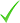Professional Course

# A-level Mathematics for Year 12 - Course 2

edX, Online
Length
7 weeks
Price
49 USD
Next course start
Start anytime See details
Delivery
Self-paced Online
Length
7 weeks
Price
49 USD
Next course start
Start anytime See details
Delivery
Self-paced Online
Visit this course's homepage on the provider's site to learn more or book!

## A-level Mathematics for Year 12 - Course 2: Calculus, Newton’s Laws and Hypothesis Testing

This course by Imperial College London is designed to help you develop the skills you need to succeed in your A-level mathematics exams.You’ll also be encouraged to consider how what you know fits into the wider mathematical world.

Over seven modules, covering an introduction to calculus, Newton’s laws and statistical hypothesis testing your initial skillset will be extended to give a clear understanding of how background knowledge underpins the A -level course.

You will investigate key topic areas to gain a deeper understanding of the skills and techniques that you can apply throughout your A-level study. These skills include:

• Fluency selecting and applying correct methods to answer with speed and efficiency
• Confidence critically assessing mathematical methods and investigating ways to apply them
• Problem solving analysing the ‘unfamiliar’ and identifying which skills and techniques you require to answer questions
• Constructing mathematical argument using mathematical tools such as diagrams, graphs, logical deduction, mathematical symbols, mathematical language, data handling, construct mathematical argument and present precisely to others
• Deep reasoning analysing and critiquing mathematical techniques, arguments, formulae and proofs to comprehend how they can be applied

## Upcoming start dates

1 start date available

#### Start anytime

• Self-paced Online
• Online
• English

## Sylabbus

### Differentiation

• The derivative of f(!) as the gradient of the tangent to the graph of " = f(!) at a general point (!,")
• Derivatives as a rate of change
• Differentiation from first principles
• Differentiating !#

#### Differentiation 2

• Differentiating !# for rational values of #
• Applying differentiation to find maxima and minima and stationary points.
• Increasing and decreasing functions.
• Gradients of tangents and normal to curves.
Integration
• The Fundamental Theorem of Calculus
• Integration as the reverse process of differentiation.
• Integrating !#
• Evaluating definite integrals and using a definite integral to find the area under a
• curve.
Mechanics
• The concept of a force
• Newton’s first, second and third laws.
• Motion in a straight line
• Drawing force diagrams
• Motion in a straight line under gravity
• Equilibrium of forces on a particle
• Pulleys and connected particles.

Probability Distributions

• Calculating probabilities.
• Discrete probability distributions
• The binomial distribution
• Calculating probabilities using the binomial distribution
• A-level Mathematics for Year 12 - Course 2 Syllabus

Probability Distributions

• The language of statistical hypothesis testing, developed through a binomial model
• Statistical hypotheses tests using the binomial distribution

## Course delivery details

This course is offered through Imperial College London, a partner institute of EdX.

2-4 hours per week

## Costs

• Verified Track -\$49
• Audit Track - Free

## Certification / Credits

### What you'll learn

• How to differentiate a number exponential functions
• How to apply differentiation to find stationary points
• How to find the equations of tangents and normals to curves
• How to model growth and decay using the exponential function
• How to manipulate expressions involving indices by using logarithms
• How to integrate an exponential function
• How to use integration to find the equation of a curve from its gradient function
• How to use integration to find the area enclosed by a curve
• How to use Newton’s laws to solve problems involving forces
• How to use the Binomial probability distribution to find the probability of a number of successes in a series of statistical trials
• How to define a statistical hypothesis test using the binomial distribution.

## Contact this provider

### Contact course provider

Fill out your details to find out more about A-level Mathematics for Year 12 - Course 2.Contact the providerGet more informationRegister your interest

Country *This site is protected by reCAPTCHA and the Google Privacy Policy and Terms of Service apply.
edX
141 Portland Street
02139 Cambridge Massachusetts

## edX

edX For Business helps leading companies upskill their labor forces by making the world’s greatest educational resources available to learners across a wide variety of in-demand fields. edX For Business delivers high-quality corporate eLearning to train and engage your employees...